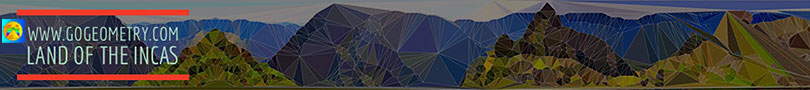# Geometry Problem 137. Orthic Triangle, Altitudes, Perpendicular, Incircle, Collinear Points, Parallelogram

In the figure below, given a triangle ABC and its orthic triangle DEF (AD, BE, and CF are the altitudes of ABC). L, M, N are the tangency points of the incircle of triangle DEF and DG and DK are perpendicular to BE and CF respectively. (1) Prove that points K, M, and L are collinear, similarly prove that points G, N, and L are collinear, (2) prove that LK, GD, and AC are parallel, similarly prove that LG, KD and AB are parallel, (3) prove that LGDK is a parallelogram, and (4) prove that GL = GM = DK. View or post a solutionHome | Geometry | Altitude | Search | Problems | 131-140 | Orthic Triangle | Email | View or post a solution